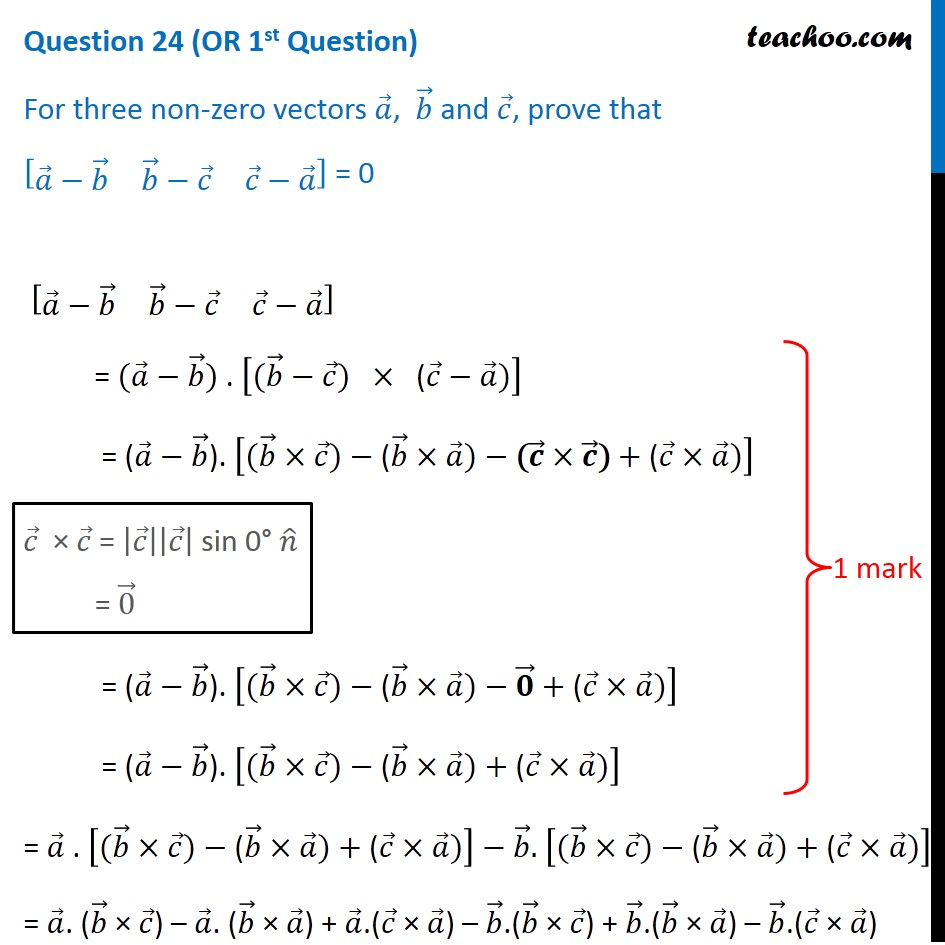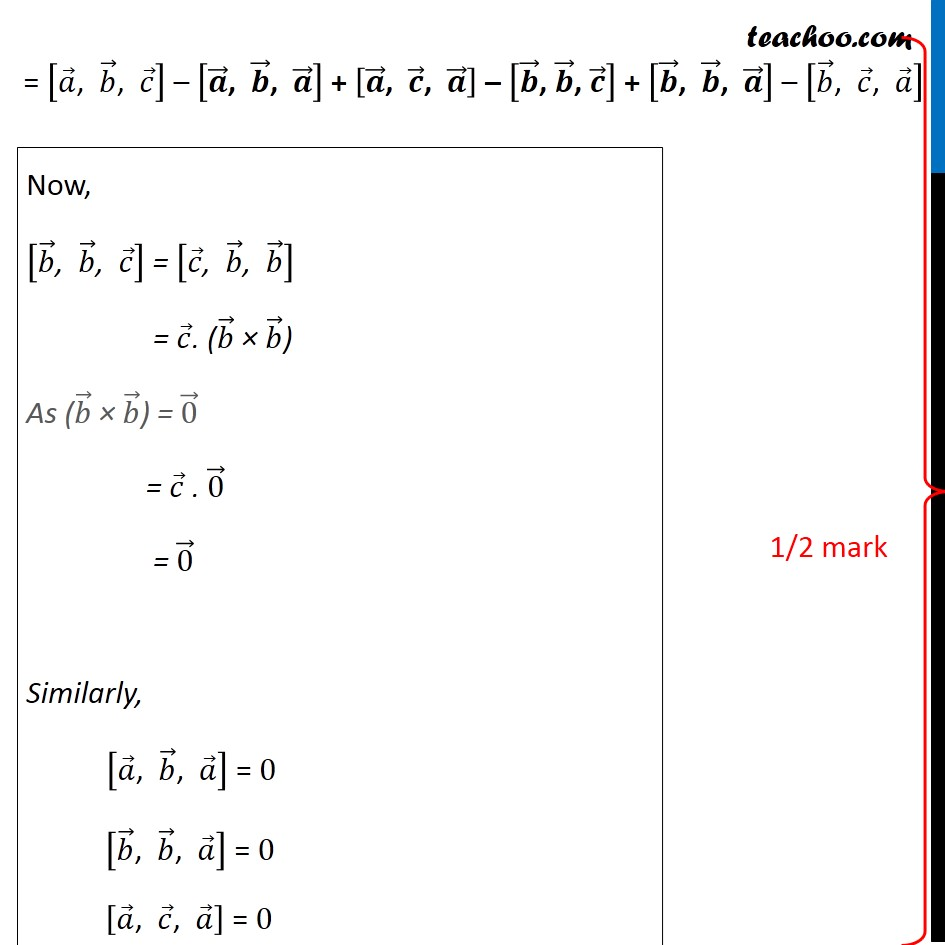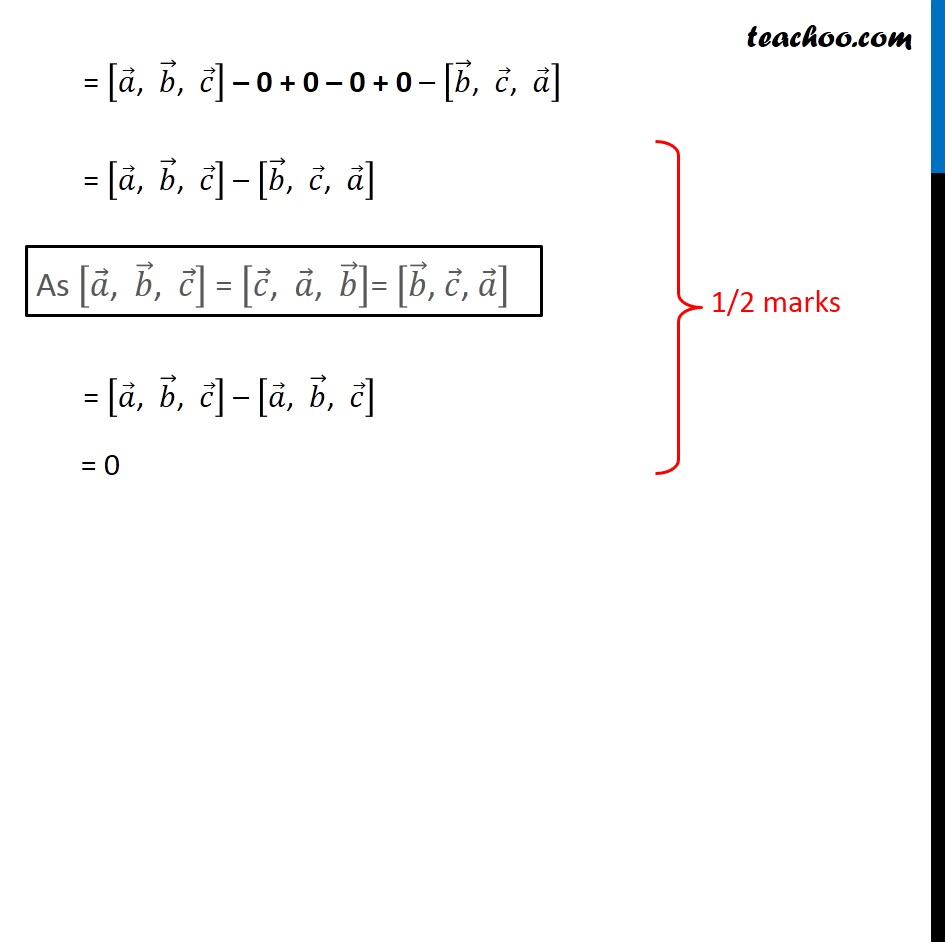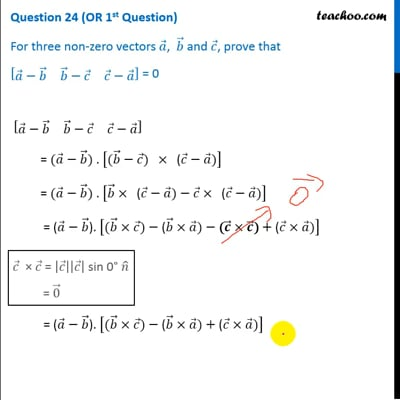CBSE Class 12 Sample Paper for 2020 Boards

Class 12
Solutions of Sample Papers and Past Year Papers - for Class 12 Boards

## [ (a - b) (b - c) (c - a)] = 0This video is only available for Teachoo black users

Note : - This is similar to Ex 10.5, 7 (Supplementary) of NCERT – Chapter 10 Class 12

Learn in your speed, with individual attention - Teachoo Maths 1-on-1 Class

### Transcript

Question 24 (OR 1st Question) For three non-zero vectors a , b and c , prove that [ (a - b) (b - c) (c - a)] = 0 [ (a - b) (b - c) (c - a)] = (a - b) (b - c) x (c - a) = (a - b) [ (b x c) - (b x a) - (c x c) + (c x a) (c x c) = [c] [c] sin 0° n = 0 = (a - b). [(b x c) - (b x a) - 0 + (c x a)] = (a - b). [ (b x c) - (b x a) - 0 + (c x a)] = a [ (b x c) - (b x a) + (c x a) -b [(b x c) - (b x a) + (c x a) = a . (b x c) -a . (b x a) + a (c x a) - b (b x c) b (b x a) - b (c x a) = [a, b, c] - [a, b, a] + [a, c, a] - [b, b, c] + [b, b, a] - [ b, c, a] now [ b, b, c] = [c, b, b] = c (b x b) as (b x b) = 0 = c .0 = 0 similarly, [a, b, a] = 0 [b, b, a] = 0 [a,c, a] = 0 = [a, b, c] - 0 + 0 - 0 + 0 - [ b, c, a] = [a, b, c] - [ b, c, a] as [a, b, c] = [c, a, b ] = - [b, c, a] = [a, b, c] - [a, b, c] = 0